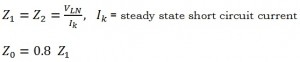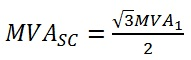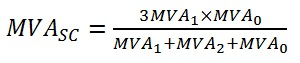# Short Circuit Faults

Can I consider the three-phase short circuit current as the worst scenario?

The one-phase or two-phase short circuit currents may be more than three-phase short circuit currents in networks in which the star point of Dy/Yy transformer is earthed (with low resistance based on standard VDE0111).

What is the load factor during short circuit?

The load factor during short circuit is about 0.15. Therefore this value shall be used to test the circuit breakers designed to protect the circuit when short circuit occurs.

What is sub-transient impedance of motors or generators?

Equipment withstand rating are based on the highest expected short-circuit current; this is called the prospective short circuit current, which usually occurs during the first half cycle of the fault. Fault currents are composed of a symmetrical AC current and most likely, an aperiodic current component known as the DC decay component.

Local generators and motors contribute in the short circuit current. This contribution in cause of DC decay component. For simplicity in the ANSI standards, the short circuit current is modelled as a constant voltage source behind a time-varying impedance. At the initial instant of the fault this impedance is known as the “sub-transient impedance”. But this changes to “transient impedance” two or three cycle into the fault. The steady state current is modelled as the machine’s synchronous impedance. In large machines, the resistance component of the machines internal impedance is ignored. There is no motor or generator contribution in steady state current.A generator short circuit current contribution is considered remote when the per unit reactance external to the generator is equal to or greater than 1.5 times the generator per unit sub-transient reactance on a common MVA base.

The slower motor has a smaller momentary short circuit current contribution than the faster motor attached to the bus.

How can I measure the positive and negative impedances of a transformer?

The positive impedance of a transformer equals the leakage impedance. It may be obtain by the usual short circuit test. Since the transformer is a static device, the leakage impedance does not change, if the phase sequence is altered from RYB to RBY. Therefore, the negative sequence impedance of transformer is the same as the positive sequence impedance.

The zero sequence impedance of the transformer depends on the winding type (star or delta) and also on the type of earth connection.

The positive and negative sequence per-unit impedances are independent of whether the sequence currents are injected into the primary or the secondary. However the zero sequence impedance will have different values, depending upon whether the sequence currents are injected into the primary or the secondary. Note that the zero sequence currents cannot be injected into the delta terminals.

What is X/R ratio?

Ratio of the system reactance to resistance. It is indicative of the rate of decay of any DC off-set in short circuit currents. A large X/R ratio corresponds to a large time constant and a slow rate of decay.

What is percentage impedance of equipment?

Manufacturers give impedance of equipment in percent on own base. The percent value is the per unit value multiplied by 100 (Z% = Zpu × 100). The expression “own base” means that the base voltage is the rated voltage of the equipment, and the base power is the rated apparent power (in VA) of the equipment. The base current and the base impedance are calculated from the base voltage and the base VA.Example – percentage impedance of a transformer

In order to determine the percentage impedance of a transformer, the secondary should be short circuited. Then increase the input voltage in primary till the current in primary reaches the Ibase. Measure the implemented voltage (V).Therefore the percentage impedance can be achieved from measured primary voltage.

How does the MVA method work to calculate the short circuit current?

The MVS method is used for solving industrial power system short circuits calculation.  Basically the MVA method is a modification of the Ohmic method in which the impedance of a circuit is the sum of the impedances of various components of the circuit. The below calculations are for three phase fault current calculation.Now consider two pieces of equipment (i.e. main network and a transformer) in series contributing in short circuit current.Now consider two pieces of equipment (i.e. a motor and a transformer) in parallel contributing in short circuit current.It can easily be recognized that series MVA combinations are exactly as resistance computed in parallel. Parallel MVA contributions are exactly as resistances computed in series.

Note that in MVA method, it does not require a common MVA base as required by per unit method. It is not necessary to convert impedances from one voltage to another as required by the ohmic method.

Can other short circuit currents rather that three phase fault be solved by MVA method?

The answer is yes. Please consider below notes in calculating fault current.

Power linesTransformersThe transformer zero sequence reactance is equal to its positive and negative reactances. The delta primary of the transformer blocks any zero sequence power following from the system and across the transformer.

Asynchronous motorsThe zero-sequence impedance of asynchronous motors may be taken as an open circuit, as the winding are usually delta or unearthed star connected (AS 3851).

VFD or convertors

If non regenerative then ignore it. If regenerative then same as motors ( ).

Synchronous generators/motors/compensatorsThe zero sequence reactance of the motor is about ½ of its positive sequence reactance.

It is also widely recognised and accepted by industries to use reactances only in calculating industrial power system short circuit, in that it would result in a higher short circuit value, perhaps by 0-3 percent in most cases.

The fault current for three phase unbalance faults are as follow.

• Three phase fault

For the calculation of balanced three-phase short-circuit, the positive sequence impedance is the only relevant impedance.• Phase to ground fault• Phase to phase fault• Phase to phase to ground faultWhat is the impact of different generator set rating and alternator base rating on the short current calculations?

Typically, generator reactances are published in per-unit values on a specified base alternator rating. Where the generator set rating differs from the alternator base rating it will be necessary to convert the per-unit values form the alternator base rating to the generator set rating.Should the tolerance given for generator reactances be implemented in short circuit calculation?

Reactances, including sub-transient, are expressed with a plus or minus tolerance of 10%, typically. Determination of the maximum current should use the worth case tolerance of minus 10%.

What would be the range of magnitude of the generator short circuit DC component?

The peak short circuit current in the first half cycle will include a DC component, the magnitude of which depends on the point in the cycle when the short circuit occurs. The DC component offsets the symmetrical current around the zero axes resulting in asymmetry. If the short occurs at the point where the voltage is at its peak, the DC component will be zero. If the short occurs at the point where the voltage is at zero, the DC component will be at its maximum, and the peak current will be almost two times the symmetrical current.

Typical per-unit values of Reactances for generators rating from 40 to 2000 kW.

 Reactance Description Symbol Range per-unit Period Sub-transient reactance Determines maximum instantaneous current and current at time Molded Case Circuit Breaker usually open Xd˝ 0.09 – 0.17 0 – 6 Cycles Transient Reactance Determines current at short time delay of circuit breakers Xd΄ 0.13 – 0.20 6 cycles to 5 sec. Synchronous reactance Determine steady state current without excitation support PMG (permanent magnet   generator) Xd 1.7 – 3.3 After 5 sec. Zero sequence reactance A factor in L-N short circuit current X0 0.06 – 0.09 Negative sequence reactance A factor in single-phas short circuit current X2 0.10 – 0.22

The sun-transient reactance of a generator set is used to calculate the maximum available short circuit current for selecting circuit breakers with adequate interrupting rating. Since nearly all of the generator impedances are reactance, addition of the DC component for the first few cycles may almost double the symmetrical value of current. Specifications that limit the sub-transient reactance (Xd˝) to 12 per-unit or less limit the voltage distortion caused by non-linear load currents. Calculation of the available short circuit current using per-unit values may require converting from the alternator base rating to the generator set base rating using the formula below.In practice the actual DC offset will likely range somewhere between zero and the maximum offset. However, for the purpose of selecting the interrupting rating of circuit breakers the assumption that the DC offset will be at maximum is a safe and conservative assumption (ISCsym ×2). Generally, Circuit breaker interrupting ratings are expressed in RMS symmetrical Amperes and are tested to achieve near maximum offset at the symmetrical current magnitude. Select breakers, then, based on the maximum allowable generator symmetrical short circuit current.

The alternator source voltage distortion induced by the harmonic (sub-cyclic) current distortion is directly related to the generator set sub-transient reactance. The higher the reactance is, the higher the voltage distortion.

The initial instantaneous current value (ISCsym) is controlled by the sub-transient reactance (Xd˝).

ISCsym = E/Xd˝

The peak current in the first half cycle will also include a d.c. component, the magnitude of which depends of the point in the cycle when the short circuit occurs. A DC component offset the symmetrical current around the Zero Axis resulting in an asymmetry. If the short occurs at point where voltage is at its peak, the DC component will be zero. If the short circuit occurs at the point where voltage is at zero, the DC component will be at its maximum, and the peak current will be almost two times the symmetrical current.

What is the definition of far-from-generator short circuit?

Far-from-generator and near-to-generator short circuits are defined as below in AS 3851.

Far-from-generator short-circuit
Short-circuit during which the magnitude of the symmetrical AC component of prospective short-circuit currents remain essentially constant.

Near-to-generator short-circuit
Short circuit to which at least on synchronous machine contributes a prospective initial symmetrical short-circuit current which is more than twice the generator’s rated current or a short circuit to which synchronous and asynchronous motors contribute more than 5% of the initial symmetrical short-circuit current (I”k) without motors.